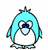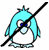Skill 1I Number Sentence for Subtraction Your student needs to understand how to make a subtraction number sentence from a picture problem that uses "cross-outs."  This is a trickier than an addition number sentence (which just counts each group) since a subtraction picture graphic requires the student to first count all of the pictures (which is the first number in the subtraction problem) and then count the pictures that are crossed-out (the second number in the problem).   Look at the picture graphic on the screen and tell me what number sentence you would write to represent the picture?